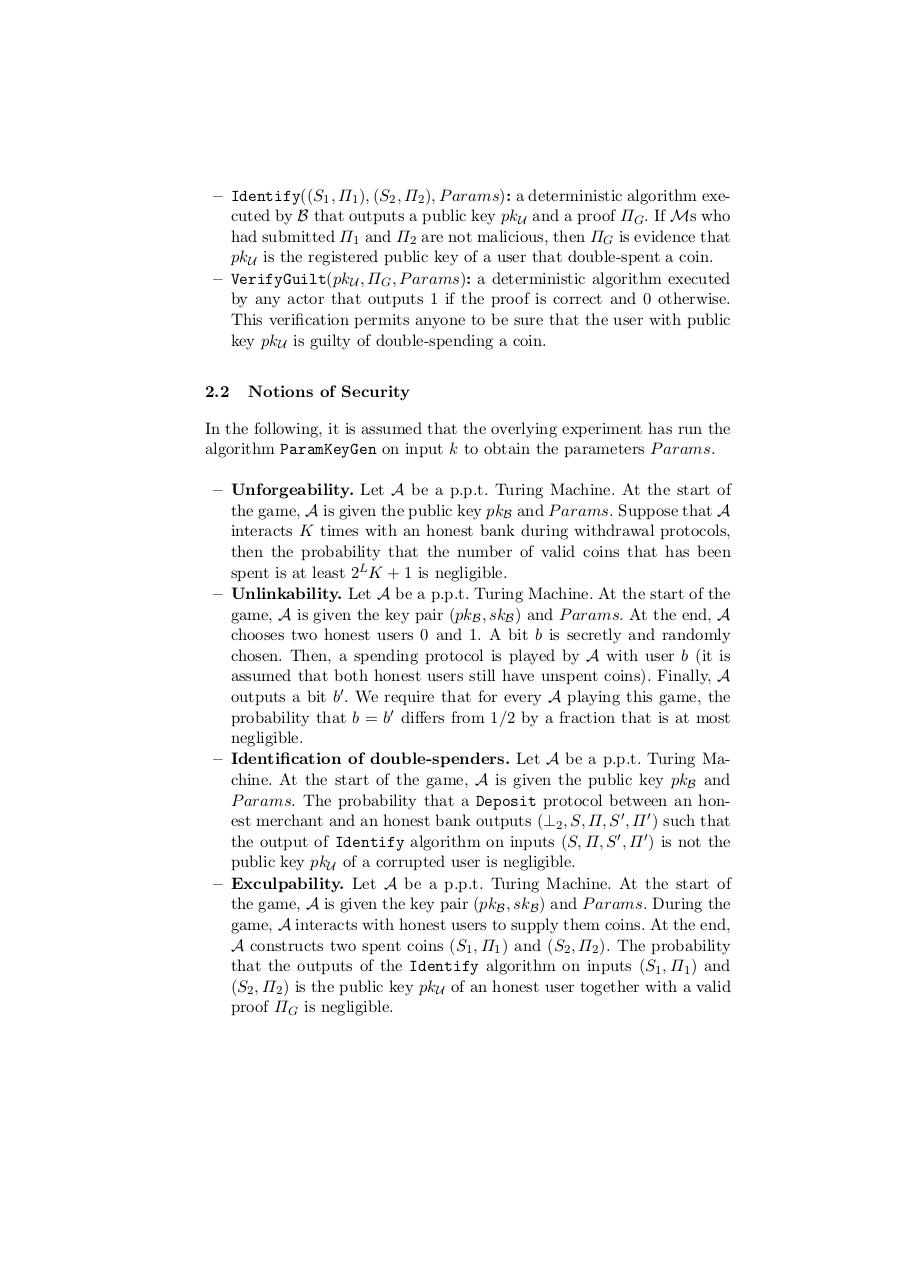# e cash satoshi for reddit.pdfPage 1 2 3 45618

#### Text preview

– Identify((S1 , Π1 ), (S2 , Π2 ), P arams): a deterministic algorithm executed by B that outputs a public key pkU and a proof ΠG . If Ms who
had submitted Π1 and Π2 are not malicious, then ΠG is evidence that
pkU is the registered public key of a user that double-spent a coin.
– VerifyGuilt(pkU , ΠG , P arams): a deterministic algorithm executed
by any actor that outputs 1 if the proof is correct and 0 otherwise.
This verification permits anyone to be sure that the user with public
key pkU is guilty of double-spending a coin.
2.2

Notions of Security

In the following, it is assumed that the overlying experiment has run the
algorithm ParamKeyGen on input k to obtain the parameters P arams.
– Unforgeability. Let A be a p.p.t. Turing Machine. At the start of
the game, A is given the public key pkB and P arams. Suppose that A
interacts K times with an honest bank during withdrawal protocols,
then the probability that the number of valid coins that has been
spent is at least 2L K + 1 is negligible.
– Unlinkability. Let A be a p.p.t. Turing Machine. At the start of the
game, A is given the key pair (pkB , skB ) and P arams. At the end, A
chooses two honest users 0 and 1. A bit b is secretly and randomly
chosen. Then, a spending protocol is played by A with user b (it is
assumed that both honest users still have unspent coins). Finally, A
outputs a bit b′ . We require that for every A playing this game, the
probability that b = b′ differs from 1/2 by a fraction that is at most
negligible.
– Identification of double-spenders. Let A be a p.p.t. Turing Machine. At the start of the game, A is given the public key pkB and
P arams. The probability that a Deposit protocol between an honest merchant and an honest bank outputs (⊥2 , S, Π, S ′ , Π ′ ) such that
the output of Identify algorithm on inputs (S, Π, S ′ , Π ′ ) is not the
public key pkU of a corrupted user is negligible.
– Exculpability. Let A be a p.p.t. Turing Machine. At the start of
the game, A is given the key pair (pkB , skB ) and P arams. During the
game, A interacts with honest users to supply them coins. At the end,
A constructs two spent coins (S1 , Π1 ) and (S2 , Π2 ). The probability
that the outputs of the Identify algorithm on inputs (S1 , Π1 ) and
(S2 , Π2 ) is the public key pkU of an honest user together with a valid
proof ΠG is negligible.